This chapter can improve student’s math skills, by referring to the Go Math Grade 3 Answer Key Chapter 1 Addition and Subtraction within 1,000 Assessment Test, and with the help of this Go Math Grade 3 Assessment Test Answer Key, students can score good marks in the examination.

Go Math Grade 3 Answer Key Chapter 1 contains all the topics of chapter 1 which helps to test the student’s knowledge. Through this assessment test, students can check their knowledge. This assessment test is also helpful for the teachers to know how much a student understood the topics.

Chapter 1: Addition and Subtraction within 1,000 Assessment Test

### Test – Page 1 – Page No. 11

Question 1.
For numbers 1a–1d, choose Yes or No to tell whether the sum is even.
a. 8 + 3
i. yes
ii. no

Explanation: As 8 + 3 = 11 which is odd number. So the answer is no.

Question 1.
b. 6 + 6
i. yes
ii. no

Explanation: As 6 + 6= 12 which is even number. So the answer is true.

Question 1.
c. 4 + 5
i. yes
ii. no

Explanation: As 4 + 5 = 9 which is odd number. So the answer is No.

Question 1.
d. 2 + 6
i. yes
ii. no

Explanation: As 2 + 6 = 8 which is even number. So the answer is Yes.

Question 2.
Select the number sentences that show the Commutative Property of Addition. Mark all that apply.
Options:
a. 9 + 7 = 16 + 0
b. 9 + 7 = 7 + 9
c. (4 + 5) + 7 = (5 + 4) + 7
d. 7 + (4 + 5) = (7 + 4) + 5

Explanation: The “Commutative Laws” say we can swap numbers over and still get the same answer when we add a + b = b+a. Therefore 9 + 7 = 7 + 9 and (4 + 5) + 7 = (5 + 4) + 7 shows the Commutative Property of Addition.

Question 3.
Select the numbers that round to 500 when rounded to the nearest hundred. Mark all that apply.
Options:
a. 438
b. 542
c. 450
d. 483
e. 567

Explanation: We know that 542, 450, and 483 are between 400 and 500 and it is closer to 500. So, 483 rounded off to the nearest hundred is 500.

Question 4.
There are 165 cars in the parking lot. Complete the chart to show 165 rounded to the nearest 10.Explanation: Rounding 165 to nearest 10 = 170.
1 hundred, 7 tens, and 0 ones =  170.### Test – Page 2 – Page No. 12

Question 5.
Write each number sentence in the box below the better estimate of the sum.
281 + 125 = ■          236 + 119 = ■
242 + 128 = ■         309 + 135 = ■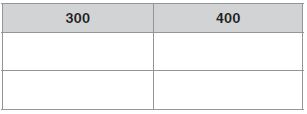281 + 125 = 300 + 100 = 400        236 + 119 = 200 + 100 = 300
242 + 128 =   200 + 100 = 300       309 + 135 =  300 + 100 = 400

Explanation: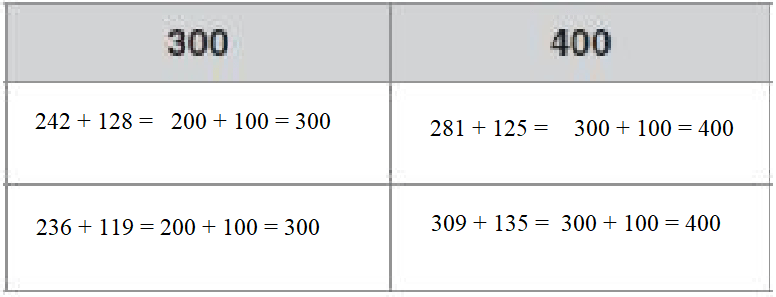Question 6.
Abby and Cruz are playing a game. Abby’s score is 586 points. Cruz’s score is 754. Abby estimates she needs about 200 points more to reach Cruz’s score. How did she estimate? Explain.

Answer: Cruz rounded the estimates points to the nearest hundred.

Explanation: Cruz rounded 586 to 600 and 754 to 800. Then she calculated the difference to estimate points = 800 – 600 = 200.

Question 7.
The table shows how many shells each person collected.For numbers 7a–7d select True or False for each statement.
a. Melba collected about 40 more shells than Pablo.
i. True
ii. False

Explanation:
Melba collected 455 shells and Pablo collected 421 shells
Difference between both = 455 – 421 = 34 which is near to 40.

Question 7.
b. Melba and Pablo collected more than 800 shells.
i. True
ii. False

Explanation: Sum of shells collected by Melba and Pablo = 455 + 421 = 876.

Question 7.
c. Amber collected about 60 fewer shells than Pablo.
i. True
ii. False

Explanation: Difference between Amber and Pablo collected shells = 421 – 382 = 39.

Question 7.
d. Amber, Melba, and Pablo collected over 1,100 shells.
i. True
ii. False

Explanation: Sum of shells collected all three = 382 + 455 + 421 = 1258.

### Test – Page 3 – Page No. 13

Question 8.
Mikio drove 58 miles on Saturday. On Sunday he drove 23 miles. How many miles did he drive on Saturday and Sunday? Explain how you solved the problem.
_____ miles

Explanation:
No of miles drove on Saturday = 58 miles
No of miles drove on Sunday = 23 miles
Total no of miles he drove on both Saturday and Sunday = 58 + 23 = 81 miles.

Question 9.
Choose the property that makes the statement true.
TheProperty of Addition describes the number sentence 17 + 1 = 1 + 17.
________

The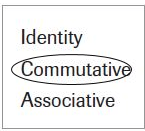Property of Addition describes the number sentence 17 + 1 = 1 + 17.

Use the table for 10–12.Question 10.
The table shows the number of students visiting the zoo each day.
How many students visited the zoo on Wednesday and Thursday?
_____ students

Explanation: No of students visited zoo on Wednesday and Thursday = 349 + 508 = 857 students.

Question 11.
How many more students visited the zoo on Wednesday than on Monday?
_____ students

Explanation: No of students visited the zoo on Wednesday than on Monday = 349 – 246 = 103.

Question 12.
How many more students visited the zoo on Monday and Tuesday than on Wednesday?
_____ students

Explanation: No of students visited the zoo on Monday and Tuesday than on Wednesday = (246+418) – 349 = 315.

### Test – Page 4 – Page No. 14

Question 13.
Help Ben find the sum.
2 4 6
3 2 1
+1 2 8
———-
695

For numbers 13a–13d choose Yes or No to tell Ben when to regroup.
a. Regroup the ones.
i. yes
ii. no

Question 13.
i. yes
ii. no

Question 13.
c. Regroup the tens.
i. yes
ii. no

Question 13.
i. yes
ii. no

Question 14.
Avery sent 58 email invitations to a party. So far, 37 people replied. How many people still need to reply? Draw jumps and label the number line to show your thinking._____ emails.

Explanation: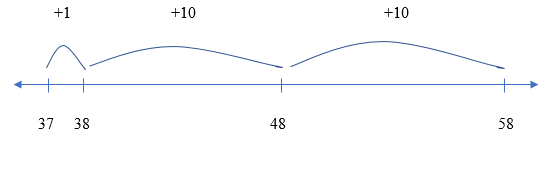Given that total 58 email invitations are sent to a party
No of people replied so far = 37
From the below figure no of people remained to reply =1 + 10 + 10
= 21 people.

Question 15.
There are 842 seats in the school auditorium. 138 seats need repairs. How many seats do not need repairs? Show your work.
_____ seats

Explanation: Total seats in school auditorium = 842
No of seats need to be repaired = 138
Therefore no of seats not required to repair = 842 – 138 = 704 seats.

Question 16.
Madison solves this problem. She says the difference is 419. Explain the mistake Madison made. What is the correct difference?
6 4 5
−2 3 6
———–
_____

Explanation: When Madison combined the tens and ones, she should have regrouped 1 ten as 10 ones to subtract 36 from 45. Then she would have 0 tens and 9 ones left. The difference is 409, not 419.

### Test – Page 5 – Page No. 15

Question 17.
Radburn School recycles aluminum cans to raise money. The third graders have collected 329 cans so far. Their goal is to collect more than 500 cans. What is the least number of cans they need to collect to reach their goal? Complete the bar model and explain how to use it to find the unknown part.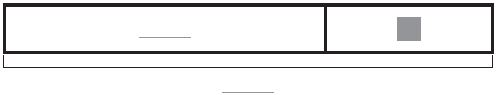_____ cans

Explanation:The given model shows a whole of 500 and a part of 329. The unknown part represents the number of cans still to be collected.
By solving using subtraction: 500 − 329 = 171. So, they need to collect 1 more can than 171, which is 172.

Question 18.
The Science Center displays 236 butterflies. The number of beetles on display is 89 less than the number of butterflies.
Part A
About how many beetles are on display at the Science Center? Explain.

Explanation:
Given that 236 butterflies have displayed in the Science Center, rounding to nearest value = 240
No of displayed beetles are 89 less than the number of butterflies, after rounding = 90
Therefore no of beetles displayed = 240 – 90 = 150.

Question 18.
Part B
How many butterflies and beetles are on display at the Science Center? Show your work.
_____ butterflies and beetles

Explanation:
Given no of butterflies = 236
No of beetles = 236 – 89 = 147
Total no of beetles and butterflies = 236 + 147 = 383.

### Test – Page 6 – Page No. 16

Question 19.
Elena used 74 + 37 = 111 to check her subtraction. Which math problem could she be checking? Mark all that apply.
Options:
a. 74 − 37 = ■
b. 111 − 74 = ■
c. 111 + 37 = ■
d. 111 − 37 = ■

Explanation: She could use either option b. 111 – 74 = 37 or option d. 111 – 37 = 74.

Question 20.
Shawn and Steve are rock hunters. The tables show the kinds of rocks they collected.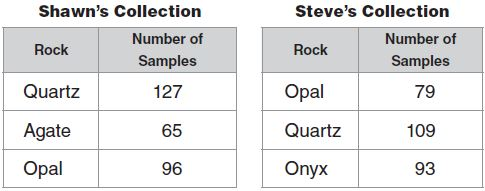Part A
Who collected more rock samples? How many did he collect? About how many more did he collect? Explain how you solved the problem.
__________

Answer: Shawn collected more rock samples which are 288.

Explanation:
Sum of rock samples collected by Shawn = (127+65+96) = 288
Sum of rock samples collected by Steve = (79 + 109 + 93) = 281
Therefore Shawn collected more rock samples compared to Steve
By subtracting 288 – 281 = 9 (after rounding) => 10
Shawn has about 10 more rock samples.

Question 20.
Part B
Shawn and Steve have the greatest number of what kind of rock? How many rocks of that kind do they have? Show your work.

Answer: Quartz rocks, 236 rocks; 127 + 109 = 236.

Explanation:
Shawn collected 127 quartz rocks where Steve collected 109 quartz rocks.
Total they both collected 236 Quartz rocks which are greatest in number compared to other types.

Conclusion:

This assessment test helps students to check their math skills. Go Math Grade 3 Chapter 10 questions are explained in detail that students can understand easily.

Scroll to Top